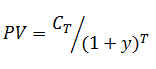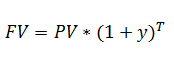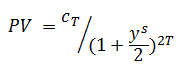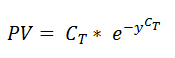# Discounting of Cash Flows

A zero coupon bond is a fixed income instrument with only one cash flow i.e. the face value of the bond which is paid to the investor at the maturity date. It is a more appropriate instrument for exemplifying the discounting of cash flows.

Present Value

The price of the zero coupon bond is determined by calculating the present value of the maturity cash flow using a discount factor or interest rate or the yield.

This can be illustrated using the formula below –Where PV indicates the present value or price of the face value or the cash flow occurring at the number of periods until maturity T and y is the yield of the zero coupon bond. This is an example of how to discount a single cash flow and in practice the instruments are made of more than one cash flow.

Future Value

The future value of the cash flow can be computed as follows –The yield becomes a useful measure for comparison between different financial instruments as it indicates the rate of return or the effective annual rate (EAR) which is more indicative of the quality of the investment rather than the dollar value itself.

Semi-annual Compounding

While dealing with yields the concept of compounding comes into picture. Compounding typically affects the number of periods until the maturity of the cash flow of which the yield is reflective. The number of periods for semi-annual compounding is twice the number of periods for annual compounding.

An example of semi-annual compounding is as follows –Compounding also can be quarterly, monthly, etc.

Continuous Compounding

There is one more type of compounding which is typically used in derivatives and that is continuous compounding.  It is the limit of the case where the number of compounding periods per year increases to infinity.

The formula for deriving the present value under the case of continuous compunding is as followsConversion from Annual to Semi-annual

An example of a conversion between an annual and semi-annual yield is as follows –The yield on the left is the annual yield and the yield on the right is the semi-annual yield. The more frequent the compounding the lesser will be the yield to maturity.

The above examples are for the zero coupon bond which has only one cash flow i.e. the face value at maturity. For a financial instrument with many cash flows the present value of each of them gets calculated based on the type of yield.

Get smart about tech at work.

As a non-technical professional, learn how software works with simple explanations of tech concepts. Learn more...

# Data Science for Finance Bundle: 43% OFF

Get our Data Science for Finance Bundle for just $29$51.